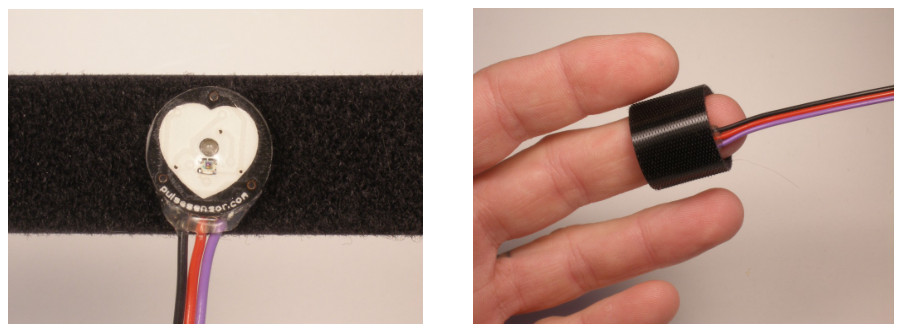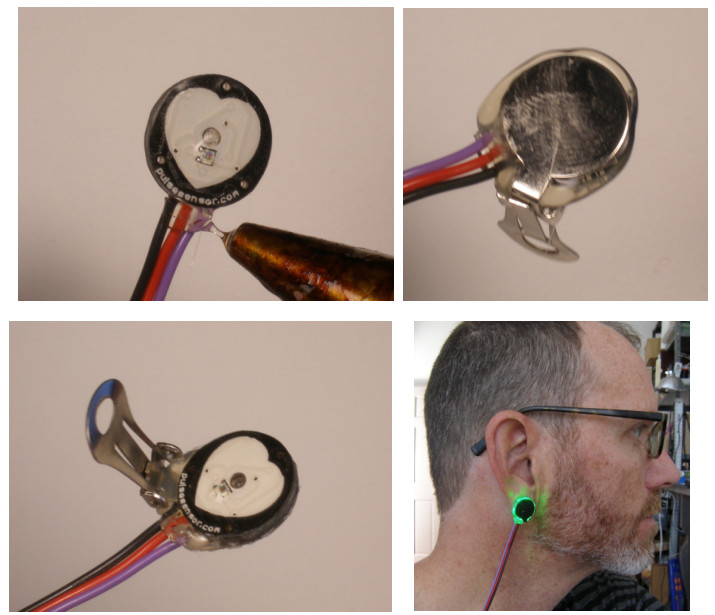# 心率传感器的使用讲解

## 心率传感器的使用，重要的是要获取到心率值，本篇文章将介绍一种采样数据处理算法——动态阈值算法，来获取心率值。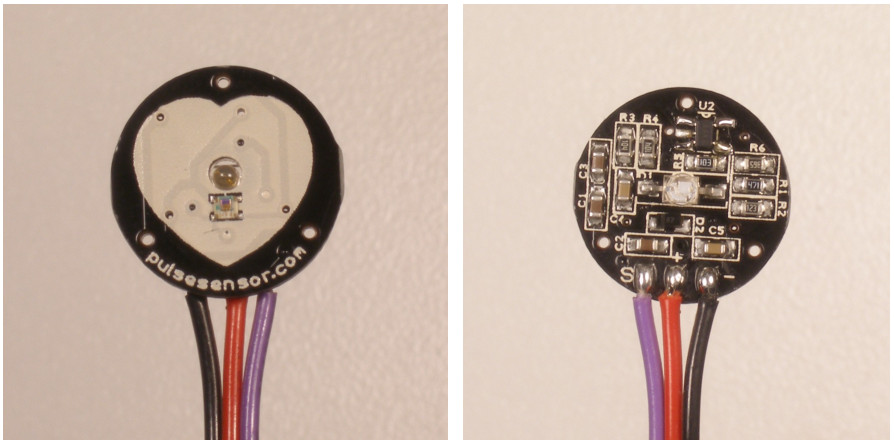### IBI和BPM

IBI： 相邻两次脉搏的时间间隔，单位：s。

BPM：心率，一分钟内的心跳次数。即BPM = 6000 / IBI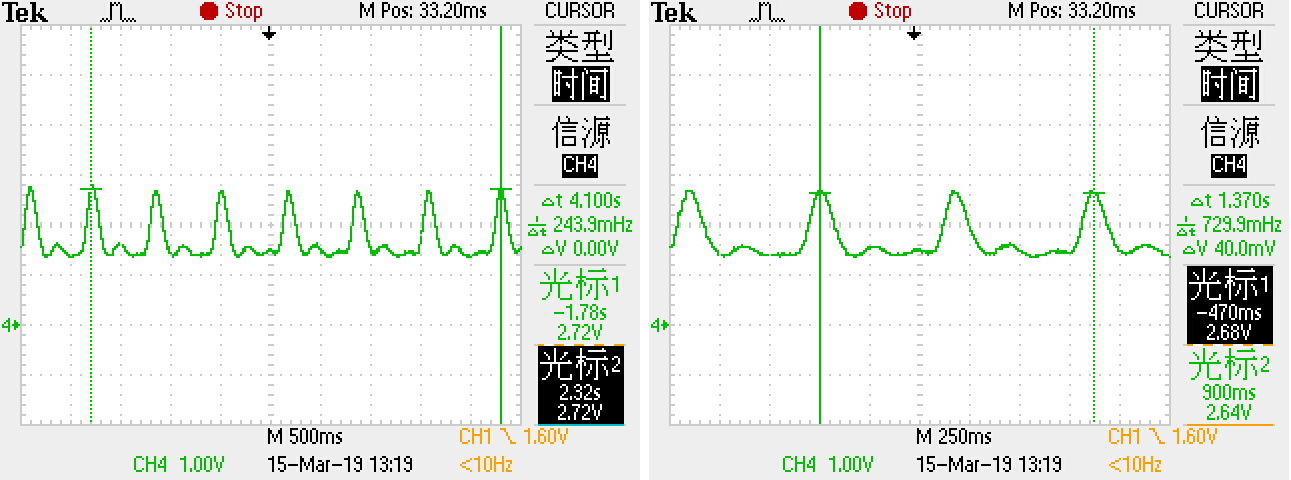### 核心操作 —— 识别一个脉搏信号

#### 问题一：阈值的选取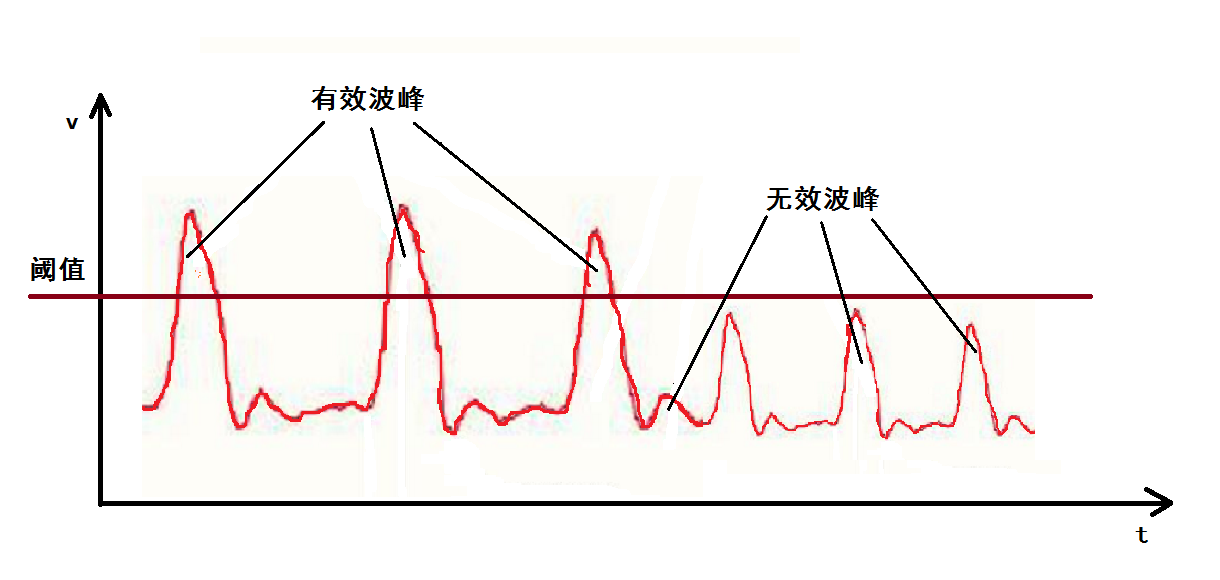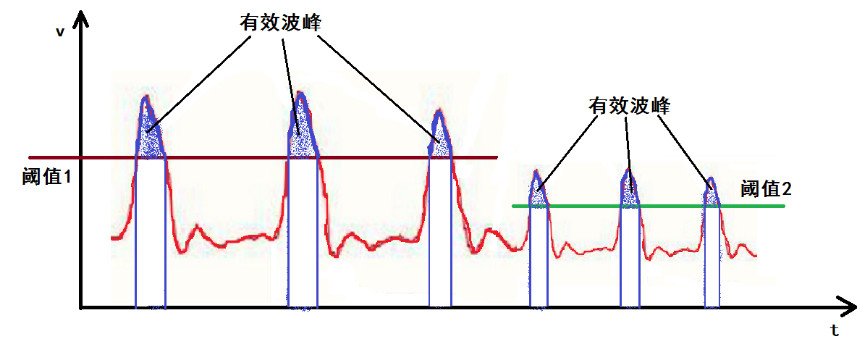#### 问题二：特征点识别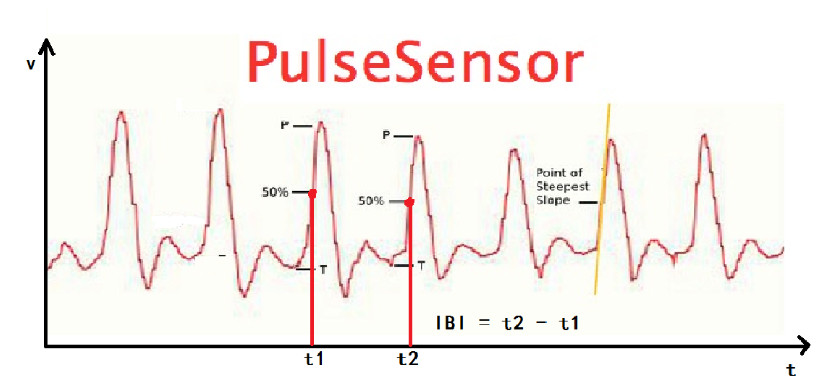### 算法整体框架与代码实现

• 缓存一个波形周期内的多次采样值，求出最大最小值，计算出振幅中间值作为信号判定阈值
• 通过把当前采样值和上一采样值与阈值作比较，寻找到「信号上升到振幅中间位置」的特征点，记录当前时间
• 寻找下一个特征点并记录时间，算出两个点的时间差值，即相邻两次脉搏的时间间隔 IBI
• 由 IBI 计算心率值 BPM

``````int main(void)
{
int i;
LED_Init();
delay_init();	    	 //延时函数初始化
UART1_Config(115200);	 	//串口初始化为9600
while(1)
{

if(idx >= DATA_SIZE)
{
idx = 0;	// 数组填满，从头再填

// 通过缓存数组获取脉冲信号的波峰、波谷值，并计算中间值作为判定参考阈值
max = Get_Array_Max(data, DATA_SIZE);
min = Get_Array_Min(data, DATA_SIZE);
mid = (max + min) / 2;
filter = (max - min) / 2;
}

PRE_PULSE = PULSE;	// 保存当前脉冲状态
PULSE = (readData > mid) ? TRUE : FALSE;  // 采样值大于中间值为有效脉冲

if(PRE_PULSE == FALSE && PULSE == TRUE)   // 寻找到“信号上升到振幅中间位置”的特征点，检测到一次有效脉搏
{
pulseCount++;
pulseCount %= 2;

if(pulseCount == 1) // 两次脉搏的第一次
{
firstTimeCount = timeCount;   // 记录第一次脉搏时间
}
if(pulseCount == 0)  // 两次脉搏的第二次
{
secondTimeCount = timeCount;  // 记录第二次脉搏时间
timeCount = 0;

if((secondTimeCount > firstTimeCount))
{
IBI = (secondTimeCount - firstTimeCount) * SAMPLE_PERIOD;	// 计算相邻两次脉搏的时间，得到 IBI
BPM = 60000 / IBI;  // 通过 IBI 得到心率值 BPM
if(BPM > 200)     //限制BPM最高显示值
BPM = 200;
if(BPM < 30)     //限制BPM最低显示值
BPM = 30;
}
}
//			printf("B%d\r\n", BPM);
printf("SIG = %d IBI = %d, BMP = %d\r\n\r\n", readData, IBI, BPM);
}
//        printf("S%d\r\n", SIG);  // 上位机S数据发送
timeCount++;  // 时间计数累加
delay_ms(SAMPLE_PERIOD);  // 延时再进行下一周期采样
if(i++ >= 50)
{
LED = !LED;
i = 0;
}
}
}
``````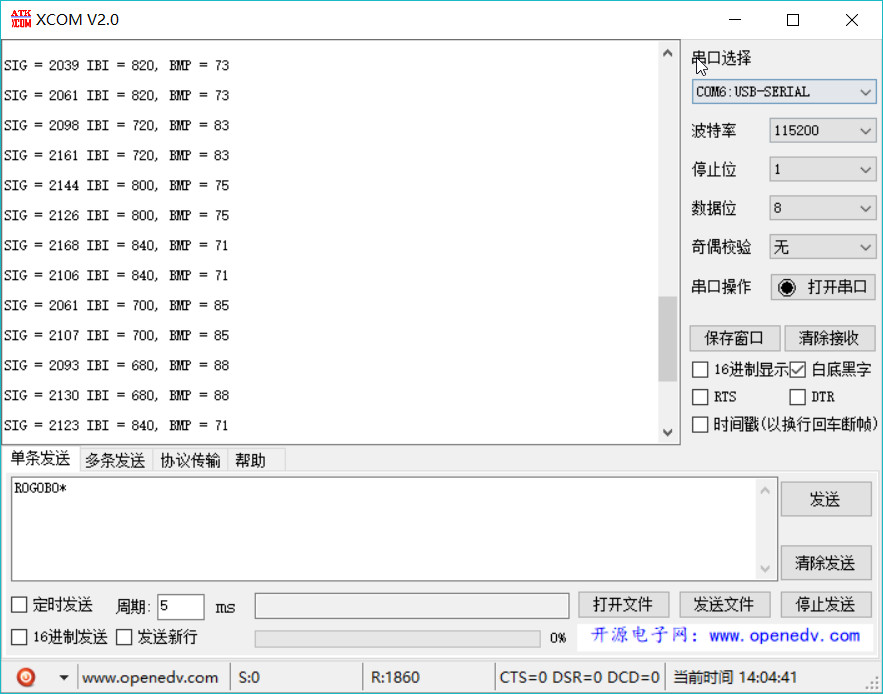• 避免手指触碰传感器背面
• 传感器与手指之间不要施加过大压力，否则会阻碍血液流动而读不到脉搏信号
• 传感器与手指之间的接触要保持稳定，按压力度的轻微变化都会影响电压值